## Artificial life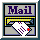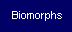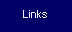Introduction to Cellular Automata
History
The Game of Life
Other Cellular Automata
Applications
Cellular Automata and Artificial Life
Emergence
Self-Reproduction
Chaos and complexity
Bibliography

 Download that text in PDF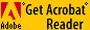----------------------------------------------------------------------------------------------------

3- Chaos and complexity

The number of possible cellular automata is potentially infinite. In this context, Wolfram wondered about the existence of cellular automata's general behaviour rules22.

S. Wolfram studied one dimensional automata, with two states and a neighbourhood of 2. He only considered as "legal" the automata that firstly eliminate any cell without neighbour, and secondly, are symmetric. There are then only 32 "legal" automata that Wolfram systematically studied.

This study showed that, according to the author, numerous cellular automata, and maybe all of them, fall into four basic classes.

Class I- evolution leads to an homogeneous state.

Class I automata (rule 36)Class II- Evolution leads to simple or periodic structures.

Class II automata (rule 40)Class III- Evolution leads to chaotic states.

Class III automata (rule 18)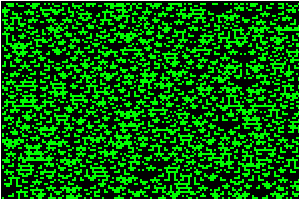Class IV- Evolution leads to complex global structures.

Class IV automata (rule 20)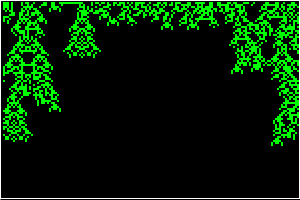According to Wolfram, cellular automata belonging to class IV generate structures that are strongly reminiscent of the game of life. Yet it is known that this cellular automaton has the computational universality property. This means that : "Cellular Automata may be viewed as computers, in which data represented by initial configurations is processed by time evolution. Computational universality implies that suitable initial configurations can specify arbitrary algorithmic procedures"23. He then puts forward the hypothesis that class IV characterizes the automata having universal computation capability. In order to let this capability emerge, the cells must be able to communicate and to transmit information. In classes I and II automata cells are to strongly interdependent to allow a useful information processing. Class III automata are characterized by a too weak cells interdependence. Most of automata belong to classes I to III. They represent 30 over the 32 Wolfram's automata. cellular automata belonging to class IV are then only to be found in a minority of cases. The cellular automata that are at the limit between classes I and II on the one hand, and class III on the other hand, are the only ones to be capable to deal with information in a useful way, and therefore are the only "interesting" ones24.

C. Langton was also interested in the existence of general classification rules of cellular automata but in a more general way. The difficulty lies into the number of possible automata. If we consider the only automata with 8 states and a neighbourhood of 5, there are 8 power 85, i.e. 832768 possible universes. Langton decided to classify the cellular automata depending on a general parameter. Theparameter is, in fact, the probability, within all possible neighbourhood configurations, that one given configuration should lead to an "active" cell, i.e. : 1 - (number of quiescent transitions/ total number of transitions) . Using this parameter to build transition rules, Langton managed to classify cellular automata. For a low value, cells quickly disappear. If the value is raised over about 0.2, cyclical or persisting structures appear. Over about 0.3, complex and unpredictable behaviours appear. Finally, over about 0.5, the multiplication of structures induces a chaotic behaviour. To a certain extent theparameter indicates the temperature of the universe of the cellular automata25.

The four following figures show examples of one dimension automata with eight states and a neighbourhood of 526.=0.1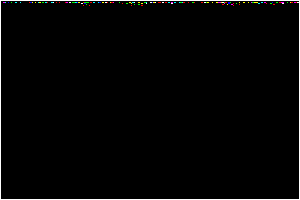=0.25=0.45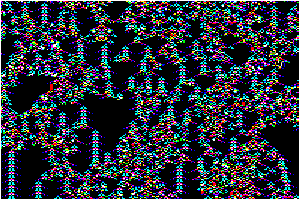=0.8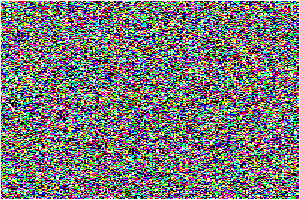We then find again Wolfram's classification, but in a more general framework. According to Langton, the automata belonging to class IV, those whoseparameter is roughly to be found between 0.3 and 0.5, are those whose ability to process information is the most important. "(...) cellular automata capable of performing non trivial computation - including universal computation - are most likely to be found in the vicinity of "phase transitions" between order and chaos (...)"27.

J.C. Heudin28 uses a parameter () that, in two dimensions cellular automata, corresponds to the number of active neighbours necessary for a cell to remain unchanged. In the game of life, this parameter value is 2. He then redefines automata with four rules :

1. R1 : the neighbourhood is inferior to: the cell dies.
2. R2 : a cell surrounded byliving cells keeps its state.
3. R3 : a cell that has+ 1 living neighbours becomes alive.
4. R4 : a cell surrounded by more than+ 1 neighbours dies.

For=1, the probabilities of rules execution are R4>R3>R2>R1. For= 2, we've got a complete inversion with R1>R2>R3>R4. Beyond 2, the order remains identical, but the rules R2, R3 and R4 are very seldom executed. Heudin then shows a fundamental change in the universe of cellular automata for= 2. It is during this deep modification of the automata properties - this phase transition - that complexity appears : "To appear [complex] needs order and a pinch of chaos. This situation occurs only at the interface of both systems, at the border who leads to chaos."29.

With the generalization of cellular automata behaviours, diversity of universal structures or the existence of life, tends to show that the laws of our Universe are precisely at the border between order and chaos. This is what Langton means in the expression : "life at the edge of chaos"30.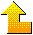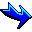22- Wolfram S., Universality and complexity in cellular automata, Physica D, 10:1-35, 1984. This text is available at  : http://www.stephenwolfram.com/publications/articles/ca/84-universality/index.html

23- Idem.

24- Gutowitz H.A., Langton C.G., Methods for designing Cellular Automata with "Interesting" Behavior, 1994. Available at : http://www.santafe.edu/~hag/interesting/interesting.html.Home - Cellular Automata - Biomorphs - Genetic Algorithms - Ants - Links